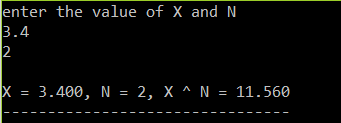# Program to evaluate Graph equation y=x^n using C Language

This is the program to evaluate graph equation y=xn. This code works with non negative integers. The following code has been written and compiled in Dev c++.

#### Code:

```#include<stdio.h>
void main(){
int count,n;
float x,y;
printf("enter the value of X and N \n");
scanf("%f%d",&x,&n);
y=1.0;
count=1;
while(count<=n){
y=y*x;
count++;
}
printf("\nX = %.3f, N = %d, X ^ N = %.3f",x,n,y);
}

```

OUTPUT:This site uses Akismet to reduce spam. Learn how your comment data is processed.